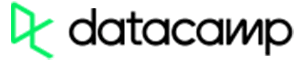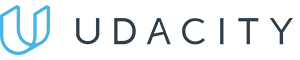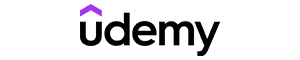Code has been added to clipboard!

# Splitting Datasets With the Sklearn train_test_split Function

Published Nov 25, 2019
Updated Jan 21, 2020

TL;DR – The train_test_split function is for splitting a single dataset for two different purposes: training and testing. The testing subset is for building your model. The testing subset is for using the model on unknown data to evaluate the performance of the model.

## What Sklearn and Model_selection are

Before discussing `train_test_split`, you should know about Sklearn (or Scikit-learn). It is a Python library that offers various features for data processing that can be used for classification, clustering, and model selection.

Model_selection is a method for setting a blueprint to analyze data and then using it to measure new data. Selecting a proper model allows you to generate accurate results when making a prediction.

To do that, you need to train your model by using a specific dataset. Then, you test the model against another dataset.

If you have one dataset, you'll need to split it by using the Sklearn `train_test_split` function first.

## What is train_test_split?

`train_test_split` is a function in Sklearn model selection for splitting data arrays into two subsets: for training data and for testing data. With this function, you don't need to divide the dataset manually.

By default, Sklearn train_test_split will make random partitions for the two subsets. However, you can also specify a random state for the operation.

### Parameters

Sklearn `test_train_split` has several parameters. A basic example of the syntax would look like this:

``train_test_split(X, y, train_size=0.*,test_size=0.*, random_state=*)``
• `X, y`. The first parameter is the dataset you're selecting to use.
• `train_size`. This parameter sets the size of the training dataset. There are three options: `None`, which is the default, `Int`, which requires the exact number of samples, and `float`, which ranges from 0.1 to 1.0.
• `test_size`. This parameter specifies the size of the testing dataset. The default state suits the training size. It will be set to 0.25 if the training size is set to default.
• `random_state`. The default mode performs a random split using `np.random`. Alternatively, you can add an integer using an exact number.Pros
• Easy to use with a learn-by-doing approach
• Offers quality content
• Gamified in-browser coding experience
• The price matches the quality
• Suitable for learners ranging from beginner to advanced
Main Features
• Free certificates of completion
• Focused on data science skills
• Flexible learning timetablePros
• Simplistic design (no unnecessary information)
• High-quality courses (even the free ones)
• Variety of features
Main Features
• Nanodegree programs
• Suitable for enterprises
• Paid Certificates of completionPros
• Easy to navigate
• No technical issues
• Seems to care about its users
Main Features
• Huge variety of courses
• 30-day refund policy
• Free certificates of completion

### The use of train_test_split

First, you need to have a dataset to split. You can start by making a list of numbers using range() like this:

``X =  list(range(15))print (X)``

Then, we add more code to make another list of square values of numbers in X:

``y = [x * x for x in X]print (y)``

Now, let's apply the `train_test_split` function. Here, we set the train size to 65% of the entire dataset. Remember to write 0.65.

``import sklearn.model_selection as model_selectionX_train, X_test, y_train, y_test = model_selection.train_test_split(X, y, train_size=0.65,test_size=0.35, random_state=101)print ("X_train: ", X_train)print ("y_train: ", y_train)print("X_test: ", X_test)print ("y_test: ", y_test)``

You can set only the `test_size` as the `train_size` will adjust accordingly. You can also set the `random_state` to 0 as shown below:

``from sklearn.model_selection import train_test_splitX_train, X_test, y_train, y_test = train_test_split(X, y, test_size = 0.3, random_state = 0)``

Note: Sklearn `train_test_split` function ignores the original sequence of numbers. After a split, they can be presented in a different order.

## Why use the Sklearn train_test_split function?

Using the same dataset for both training and testing leaves room for miscalculations, thus increases the chances of inaccurate predictions.

The `train_test_split` function allows you to break a dataset with ease while pursuing an ideal model. Also, keep in mind that your model should not be overfitting or underfitting.

### Overfitting and underfitting

Overfitting is a situation when a model shows almost perfect accuracy when handling training data. This situation happens when the model has a complex set of rules. When a model is overfitting, it can be inaccurate when handling new data.

Underfitting is when a model doesn't fit the training data due to sets of rules that are too simple. You can't rely on an underfitting model to make an accurate prediction.

## Train_test_split: useful tips

• Unless specified to use `random_state` function, `train_test_split` will split arrays into random subsets.
• The ideal split is said to be 80:20 for training and testing. You may need to adjust it depending on the size of the dataset and parameter complexity.

Days
Hours
Minutes
Seconds